Next: Definitions Up: Basic Number Theory Previous: Basic Number Theory   Contents

## Axioms

• Weak Mathematical Induction: If the following two things can be shown about a proposition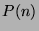:
• Base Case: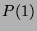is true.
• Induction Step: Ifis true, then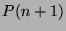is true.
thenis true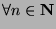.
• Strong Mathematical Induction: If the following two things can be shown about a proposition:
• Base Case:is true.
• Induction Step: If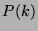is true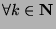,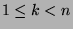, thenis true.
thenis true.
• Pigeonhole Principle: If there are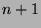pigeons and they are put in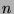pigeonholes, then there is a pigeonhole that contains at least two pigeons.
• Well Ordering Principle (WOP): If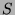is a non-empty set that is a subset of the positive integers, thenhas a least element.
• Transitivity: If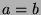and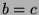, then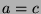.
• Substitution: If, then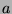can be substituted for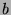in any statement without changing the veracity(truthfulness) of the statement.
• Distributive Property: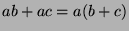.

Gregory Stoll 2000-04-08